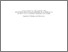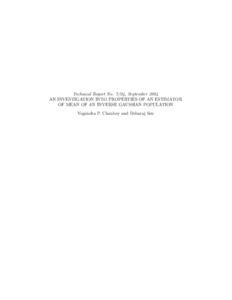Title:

# An Investigation into Properties of an Estimator of Mean of an Inverse Gaussian Population

Chaubey, Yogendra P. and Sen, Debaraj (2004) An Investigation into Properties of an Estimator of Mean of an Inverse Gaussian Population. Technical Report. Concordia University. Department of Mathematics & Statistics, Montreal, Quebec.Preview
Text (application/pdf)
7_04_Chaubey_Sen.pdf - Published Version
301kB

## Abstract

This paper deals with preliminary test estimation for mean of an inverse Gaussian population. Preliminary test estimator has been shown to provide large gains in efficiency, especially around a neighbourhood of the prior guessed value of the parameter, for many distributions including exponential and normal, however, this has not been explored for the inverse Gaussian family of distributions. Owing to diverse applications of the inverse Gaussian model for non-negative and positively skewed data, the investigation considered here makes an important contribution in the area of preliminary test estimation. We consider both the cases of known and unknown dispersion parameters and demonstrate similar conclusions as obtained in the case of Gaussian populations in terms of the efficiency of the resulting estimator.

Divisions: Concordia University > Faculty of Arts and Science > Mathematics and Statistics Monograph (Technical Report) Chaubey, Yogendra P. and Sen, Debaraj Department of Mathematics & Statistics. Technical Report No. 7/04. Concordia University. Depatment of Mathematics & Statistics Concordia University September 2004 Minimum mean square error, preliminary test estimator, inverse Gaussian population, relative bias, relative mean square error 6649 DIANE MICHAUD 02 Jun 2010 16:14 18 Jan 2018 17:29

## References:

Arnold, J.C. and Katti, S.K. (1972). An application of the Rao-Blackwell theorem in preliminary test estimators , J. of Multivariate Analysis 2, 236-238.

Bancroft, T.A. (1944). On baises in estimation due to the use of preliminary tests of significance , Annals of Math. Stat., 15, 190-204.

Bancroft, T.A. (1964). Analysis and inference for incompletely specified models involving the use of preliminary tests of significance , Biometrics, 20 427-442.

Bock, M.E., Yancey, T.A. and Judge G.G. (1973). The Statistical consequences of preliminary test estimators in regression , J. of American Statistical Association, 68, 109-116.

Folks, J.L., and Chhikara, R.S. (1978). The inverse Gaussian distribution and its statistical application-a review, J. Roy. Statist. Soc., 40, 263-289.

Chhikara, R.S., and Folks, J.L. (1989). The inverse Gaussian distribution; Theory, Methodology and applications, Marcel Deckker, New York.

Ghosh, M. and Sinha, B. K. (1988). Empirical and hierarchical Bayes competitors of preliminary test estimators in two sample problems , J. Multivariate Anal., 27, 206–227.

Han, C.P. (1978). Nonnegative and preliminary test estimators of variance components , J. of American Statistical Association, 73, 855-858.

Huntsberger, D.V. (1954). A generalization of a preliminary testing procedure
for pooling data , J. of American Statistical Association, 734-744.

Pandey, B. N. (1997). Testimator of the scale parameter of the exponential distribution using linex loss function, Comm. Statist.– Theory Methods
26, 2191–2202.

Pandey, B. N. and Malik, H. J. (1990). Testing for the mean of the inverse Gaussian distribution and adaptive estimation of parameters, Comm. Statist.- Theory Methods 19, 629–637.

Pandey, B. N., Malik, H. J. and Dubey, P. K. (1995). Bayesian shrinkage estimators for a measure of dispersion of an inverse Gaussian distribution,
Comm. Statist. Theory Methods 24, 2261–2270.

Pandey, B. N. and Srivastava, A. K. (2001) Estimation of variance using asymmetric loss function, IAPQR Trans. 26, 109–123.

Paul, A.E. (1950). On a preliminary test for pooling mean squares in the analysis of variance , Ann. Math. Statist., 21, 539-556.

Seshadri, V. (1998). The Inverse Gaussian Distribution: Statistical Theory and Applications. Springer Verlag, New York.

Tweedie, M.C.K. (1957a). Statistical properties of inverse Gaussian distributions-I , Ann. Math. Statist., 28, 362-377.

Tweedie, M.C.K. (1957b). Statistical properties of inverse Gaussian distributions-II , Ann. Math. Statist., 28, 696-705.

Whitmore, G.A. and Yalovsky, M. (1978). A normalizing logarithmic
transformation for inverse Gaussian random variables, Technometrics, 20,
207-208.

Yancey, T.A., Judge, G.G. and Bohrer, R. (1989). Sampling performance of some joint one-sided preliminary test estimators under squared error loss , Econometica, 57, 1221-1228.
All items in Spectrum are protected by copyright, with all rights reserved. The use of items is governed by Spectrum's terms of access.

Repository Staff Only: item control pageResearch related to the current document (at the CORE website)
Back to top